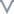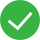# 聚合函数

### 聚合函数的作用

#### 示例

``````COUNTD([订单编号])
``````

ATTR ATTR(expression) 属性值。如果给定表达式对于组中的所有行仅具有单个相同值，则返回该表达式的值，否则返回Null。示例: ATTR(“ab”) = “ab”，ATTR([field])
AVG AVG(expression) 平均聚合。返回表达式中所有值的平均值。示例: AVG([成本])
COUNT COUNT(expression) 计数聚合。返回组中的记录数。示例: COUNT([客户名称])
COUNTD COUNTD(expression) 去重计数聚合。返回组中不同记录的数量。示例: COUNTD([产品名称])
MAX MAX(expression) 最大值聚合。返回表达式在所有记录中的最大值。示例: MAX([访问量])
MEDIAN MEDIAN(expression) 中位聚合。返回表达式在所有记录中的中位数。示例: MEDIAN([利润])
MIN MIN(expression) 最小值聚合。返回表达式在所有记录中的最小值。示例: MIN([访问量])
PERCENTILE PERCENTILE(expression, number) 百分位聚合。从给定表达式返回与指定数字对应的百分位处的值。示例: PERCENTILE([利润], 75)
STDEV STDEV(expression) 样本标准差。基于群体样本返回给定表达式中所有值的统计标准差。示例: STDEV([销售额])
STDEVP STDEVP(expression) 总体标准差。基于有偏差群体返回给定表达式中所有值的统计标准差。示例: STDEVP([销售额])
SUM SUM(expression) 求和聚合。返回表达式中所有值的总计。示例: SUM([销售额])
VAR VAR(expression) 样本方差。基于群体样本返回给定表达式中所有值的统计方差。示例: VAR([销售额])
VARP VARP(expression) 总体方差。对整个群体返回给定表达式中所有值的统计方差。示例: VARP([销售额])
GROUP_CONCAT_DISTINCT GROUP_CONCAT_DISTINCT(expression, Delimiter) 返回表达式中所有值的去重拼接结果。

## 求和聚合:SUM()

### 示例

SUM([销售额]) ，返回销售额的总计

## 平均聚合:AVG()

### 示例

AVG([销售额]) ： 返回销售额的平均值

## 计数聚合:COUNT()

### 示例

COUNT([客户名称]) 返回客户的总数

• greenplum
• oracle
• sqlserver
• postgresql
• spark
• vertica
• influxdb
• impala

• greenplum
• oracle
• sqlserver
• postgresql
• spark
• vertica
• influxdb
• impala

## 属性值聚合:ATTR(表达式)

### 去重拼接:GROUP_CONCAT_DISTINCT(表达式, 分隔符)

GROUP_CONCAT_DISTINCT(表达式, 分隔符)根本没帮助文档较差文档一般文档不错文档很好

#### 更多建议

0/200